# Chapter 21 Electric Current and DirectCurrent Circuits Current

• Slides: 40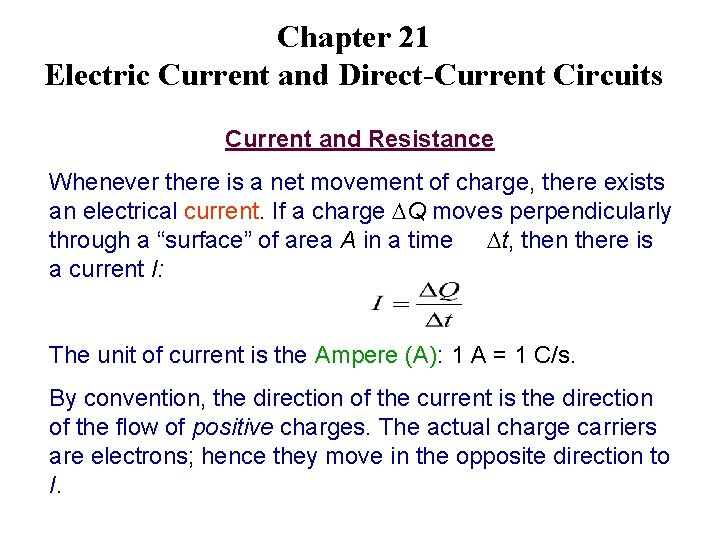Chapter 21 Electric Current and Direct-Current Circuits Current and Resistance Whenever there is a net movement of charge, there exists an electrical current. If a charge Q moves perpendicularly through a “surface” of area A in a time t, then there is a current I: The unit of current is the Ampere (A): 1 A = 1 C/s. By convention, the direction of the current is the direction of the flow of positive charges. The actual charge carriers are electrons; hence they move in the opposite direction to I.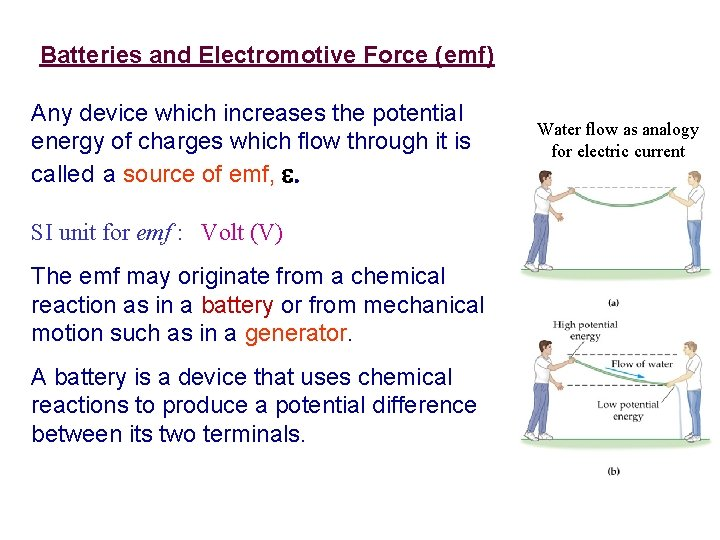Batteries and Electromotive Force (emf) Any device which increases the potential energy of charges which flow through it is called a source of emf, e. SI unit for emf : Volt (V) The emf may originate from a chemical reaction as in a battery or from mechanical motion such as in a generator. A battery is a device that uses chemical reactions to produce a potential difference between its two terminals. Water flow as analogy for electric current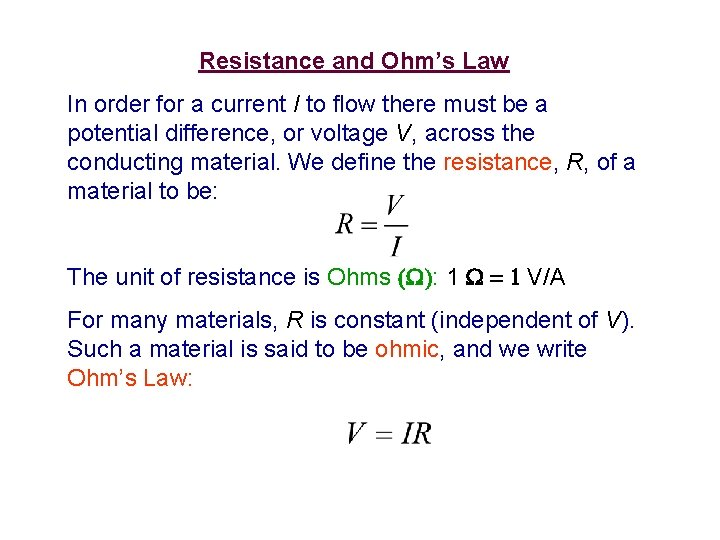Resistance and Ohm’s Law In order for a current I to flow there must be a potential difference, or voltage V, across the conducting material. We define the resistance, R, of a material to be: The unit of resistance is Ohms (W): 1 W = 1 V/A For many materials, R is constant (independent of V). Such a material is said to be ohmic, and we write Ohm’s Law: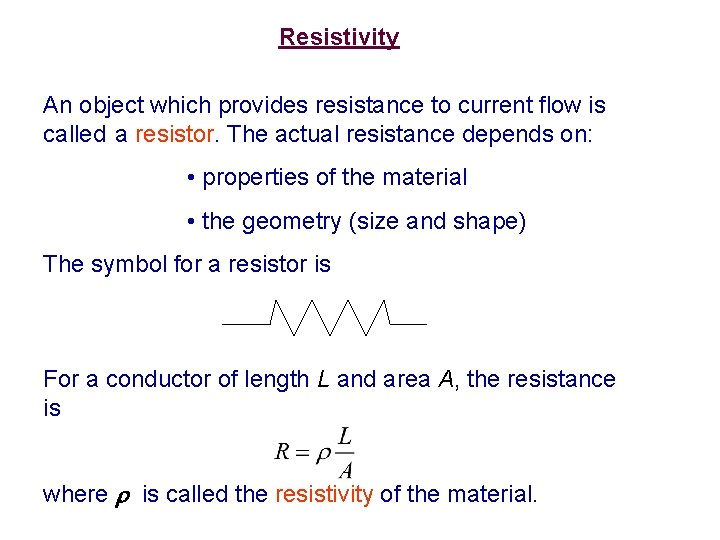Resistivity An object which provides resistance to current flow is called a resistor. The actual resistance depends on: • properties of the material • the geometry (size and shape) The symbol for a resistor is For a conductor of length L and area A, the resistance is where is called the resistivity of the material.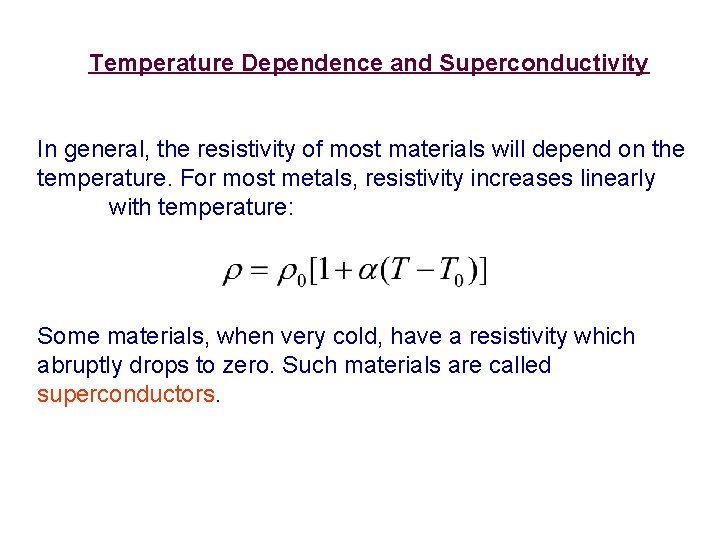Temperature Dependence and Superconductivity In general, the resistivity of most materials will depend on the temperature. For most metals, resistivity increases linearly with temperature: Some materials, when very cold, have a resistivity which abruptly drops to zero. Such materials are called superconductors.A bird lands on a bare copper wire carrying a current of 32 A. The wire is 8 gauge, which means that its cross-sectional area is 0. 13 cm 2. (a) Find the difference in potential between the bird’s feet, assuming they are separated by a distance of 6. 0 cm. (b) Will your answer to part (a) increase or decrease if the separation between the bird’s feet increases?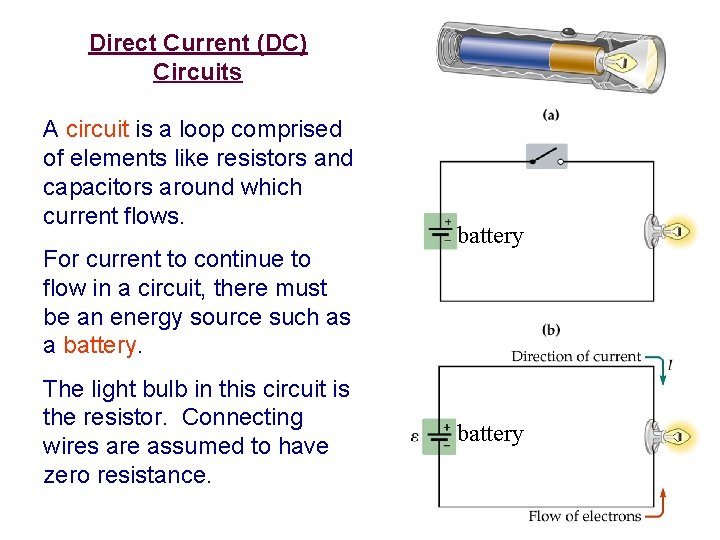Direct Current (DC) Circuits A circuit is a loop comprised of elements like resistors and capacitors around which current flows. For current to continue to flow in a circuit, there must be an energy source such as a battery. The light bulb in this circuit is the resistor. Connecting wires are assumed to have zero resistance. battery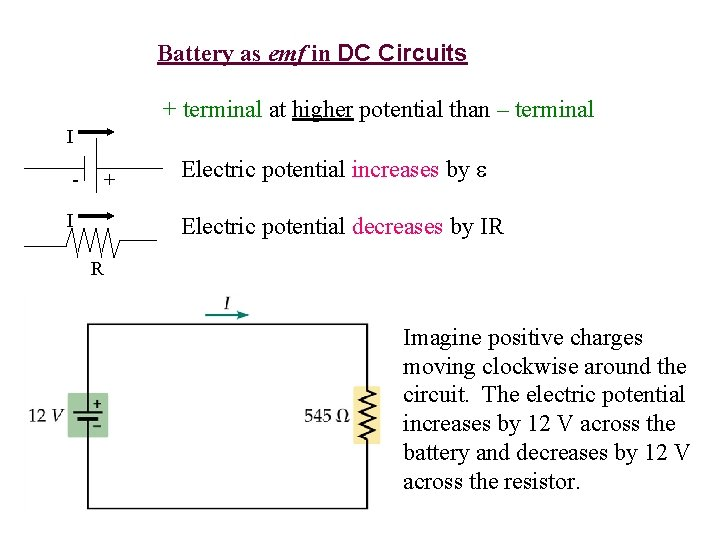Battery as emf in DC Circuits + terminal at higher potential than – terminal I - + I Electric potential increases by Electric potential decreases by IR R Imagine positive charges moving clockwise around the circuit. The electric potential increases by 12 V across the battery and decreases by 12 V across the resistor.Energy and Power in Electric Circuits Resistance is like an internal friction; energy is dissipated. The energy dissipated per unit time is the power P: P = U/ t =(DQ/Dt)V = IV SI unit: watt, W Using Ohm’s Law, V=IR, power can be rewritten as: P = I 2 R = V 2/R Energy Usage: 1 kilowatt-hour = (1000 W)(3600 s) = (1000 J/s)(3600 s) = 3. 6 106 J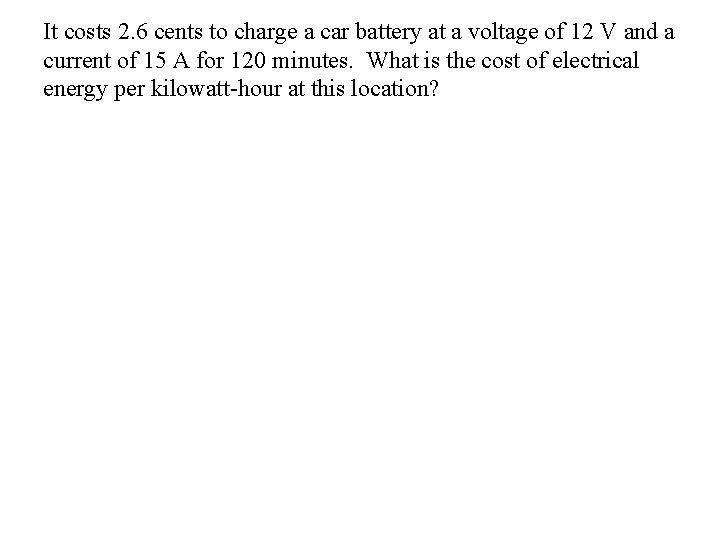It costs 2. 6 cents to charge a car battery at a voltage of 12 V and a current of 15 A for 120 minutes. What is the cost of electrical energy per kilowatt-hour at this location?A 75 -W light bulb operates on a potential difference of 95 V. Find the current in the bulb and its resistance.Resistors in Series and Parallel Any two circuit elements can be combined in two different ways: • in series - with one right after the other; the same current must flow through both elements. R 1 R 2 Series Combination R 1 • in parallel – connected across the same potential difference; the current is divided into two paths. R 2 Parallel CombinationEquivalent Resistance a R 1 R 2 R 1 b Series Combination The current I is the same in both resistors, so the voltage Vba must satisfy: Vba= IR 1 + IR 2 = I(R 1 + R 2) Req = R 1+ R 2 a R 2 b Parallel Combination The current may be different in each resistor, but the voltage Vba is the same across each resistor and the total current is conserved: I = I 1 + I 2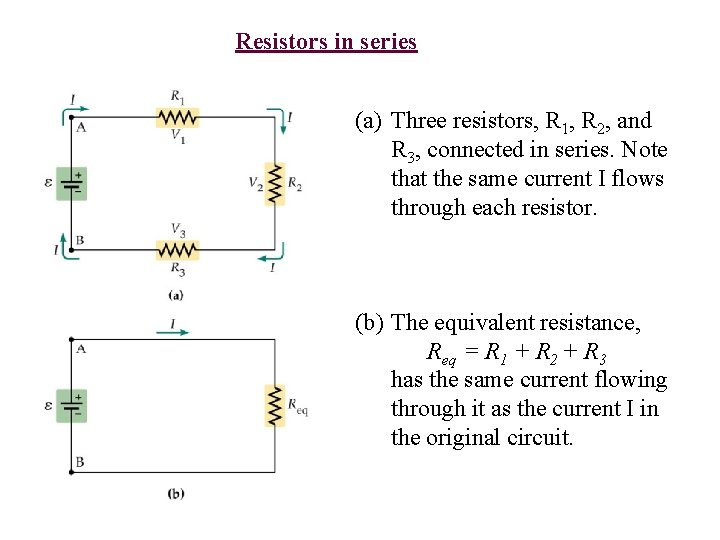Resistors in series (a) Three resistors, R 1, R 2, and R 3, connected in series. Note that the same current I flows through each resistor. (b) The equivalent resistance, Req = R 1 + R 2 + R 3 has the same current flowing through it as the current I in the original circuit.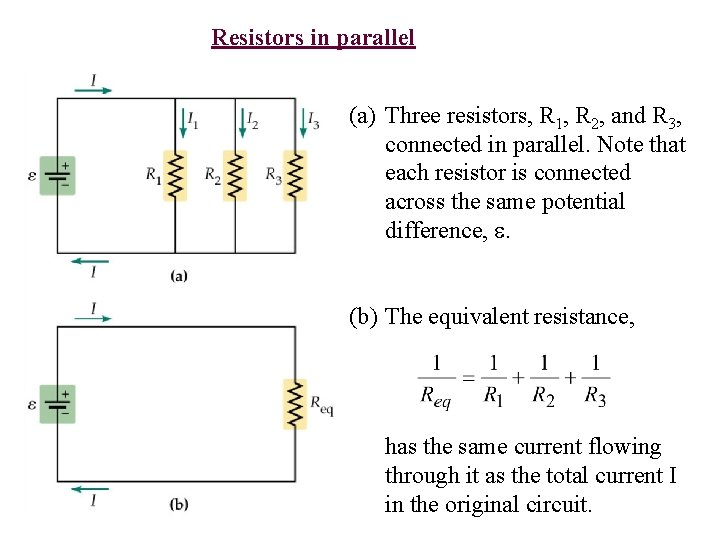Resistors in parallel (a) Three resistors, R 1, R 2, and R 3, connected in parallel. Note that each resistor is connected across the same potential difference, . (b) The equivalent resistance, has the same current flowing through it as the total current I in the original circuit.Conceptual Question Consider the circuit shown in the figure, in which three lights, each with a resistance R, are connected in parallel. What happens to the intensity of light 3 when the switch is closed? What happens to the intensities of lights 1 and 2?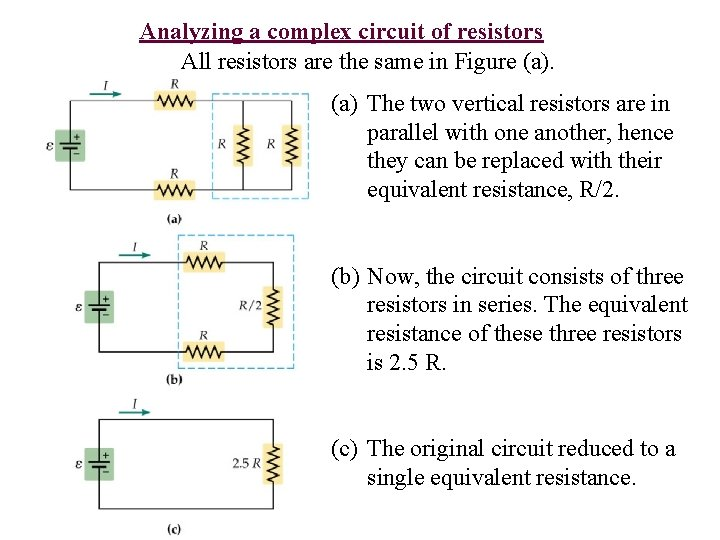Analyzing a complex circuit of resistors All resistors are the same in Figure (a) The two vertical resistors are in parallel with one another, hence they can be replaced with their equivalent resistance, R/2. (b) Now, the circuit consists of three resistors in series. The equivalent resistance of these three resistors is 2. 5 R. (c) The original circuit reduced to a single equivalent resistance.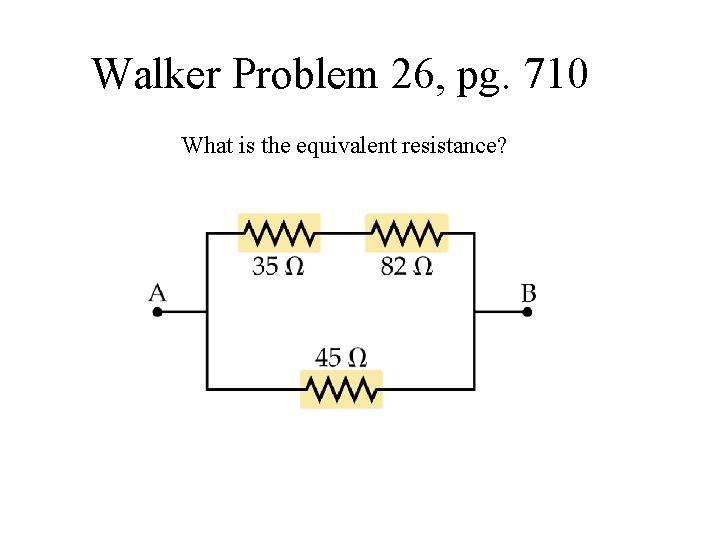Walker Problem 26, pg. 710 What is the equivalent resistance?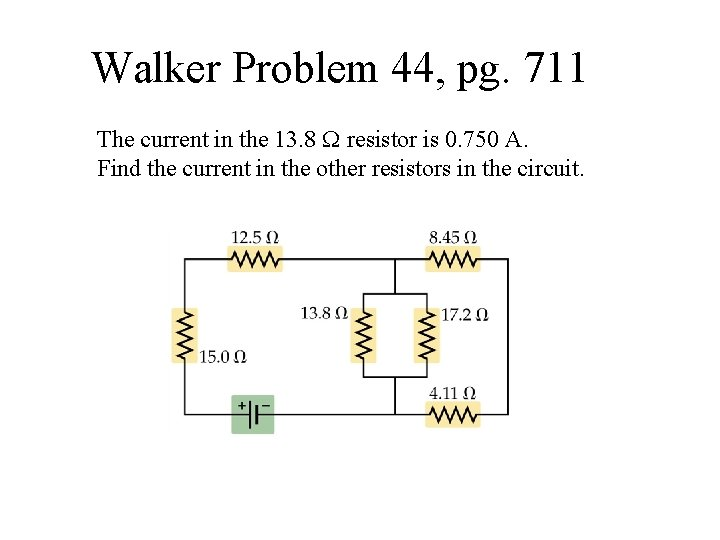Walker Problem 44, pg. 711 The current in the 13. 8 resistor is 0. 750 A. Find the current in the other resistors in the circuit.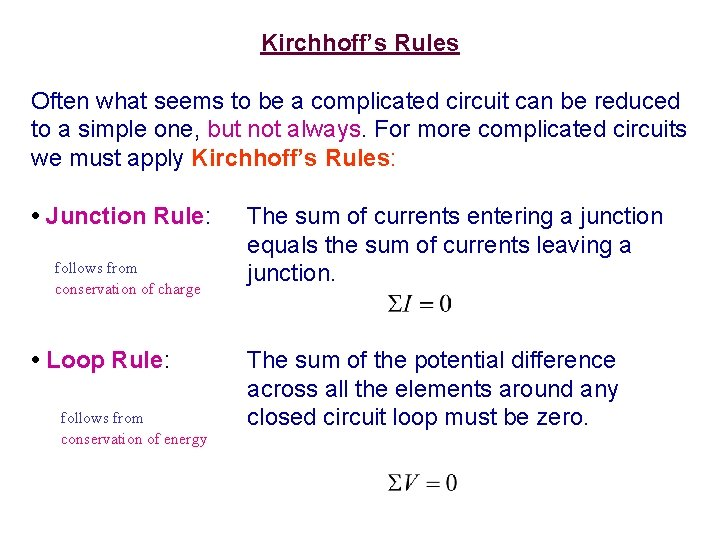Kirchhoff’s Rules Often what seems to be a complicated circuit can be reduced to a simple one, but not always. For more complicated circuits we must apply Kirchhoff’s Rules: • Junction Rule: follows from conservation of charge • Loop Rule: follows from conservation of energy The sum of currents entering a junction equals the sum of currents leaving a junction. The sum of the potential difference across all the elements around any closed circuit loop must be zero.Kirchhoff’s junction rule states that the sum of the currents entering a junction must equal the sum of the currents leaving the junction. In this case, for the junction labeled A: I 1 = I 2 + I 3 or I 1 – I 2 – I 3 = 0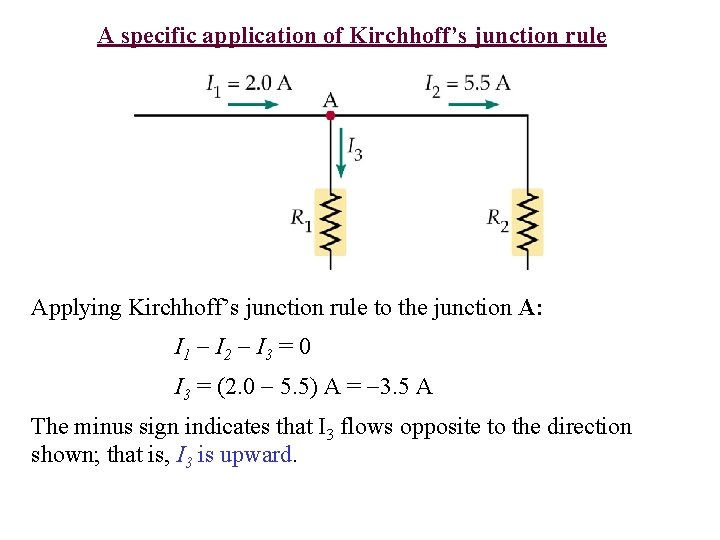A specific application of Kirchhoff’s junction rule Applying Kirchhoff’s junction rule to the junction A: I 1 I 2 I 3 = 0 I 3 = (2. 0 5. 5) A = 3. 5 A The minus sign indicates that I 3 flows opposite to the direction shown; that is, I 3 is upward.Kirchhoff’s loop rule states that as one moves around a closed loop in a circuit the algebraic sum of all potential differences must be zero. The electric potential: • increases as one moves from the minus to the plus plate of a battery • decreases as one moves through a resistor in the direction of the current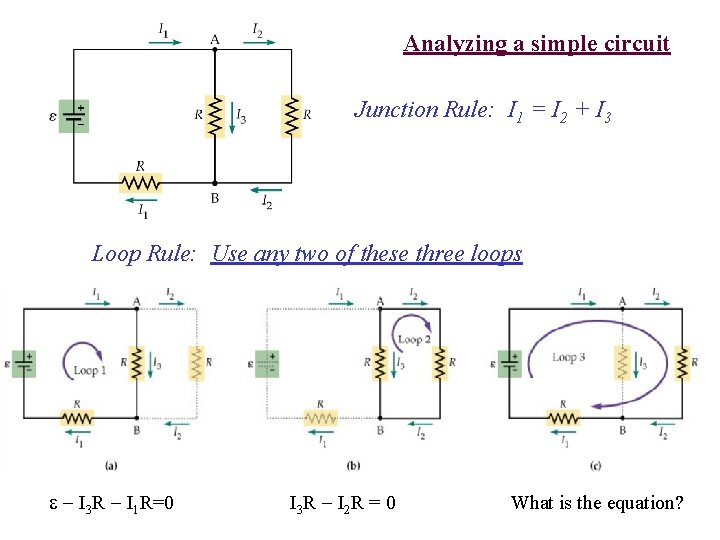Analyzing a simple circuit Junction Rule: I 1 = I 2 + I 3 Loop Rule: Use any two of these three loops I 3 R I 1 R=0 I 3 R I 2 R = 0 What is the equation?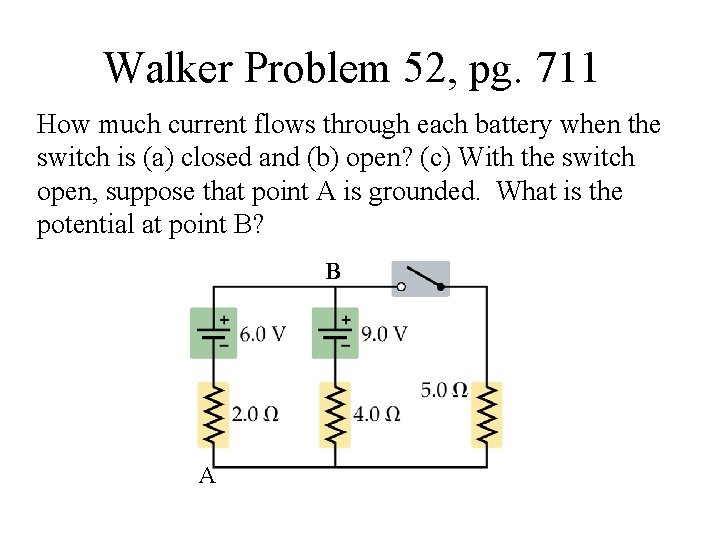Walker Problem 52, pg. 711 How much current flows through each battery when the switch is (a) closed and (b) open? (c) With the switch open, suppose that point A is grounded. What is the potential at point B? B A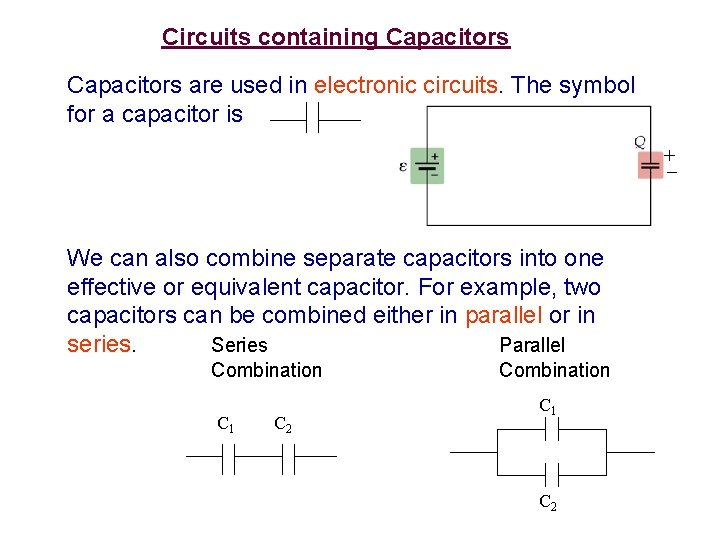Circuits containing Capacitors are used in electronic circuits. The symbol for a capacitor is + We can also combine separate capacitors into one effective or equivalent capacitor. For example, two capacitors can be combined either in parallel or in series. Series Parallel Combination C 1 C 2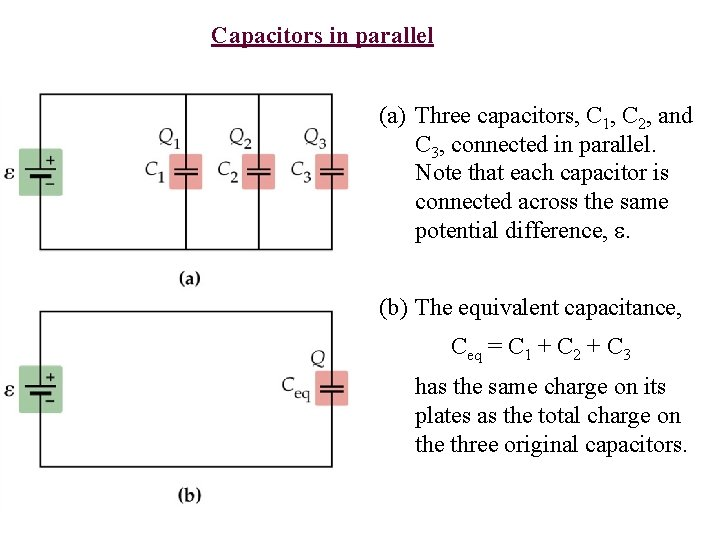Capacitors in parallel (a) Three capacitors, C 1, C 2, and C 3, connected in parallel. Note that each capacitor is connected across the same potential difference, . (b) The equivalent capacitance, Ceq = C 1 + C 2 + C 3 has the same charge on its plates as the total charge on the three original capacitors.Capacitors in series (a) Three capacitors, C 1, C 2, and C 3, connected in series. Note that each capacitor has the same magnitude of charge on its plates. (b) The equivalent capacitance, has the same charge as the original capacitors.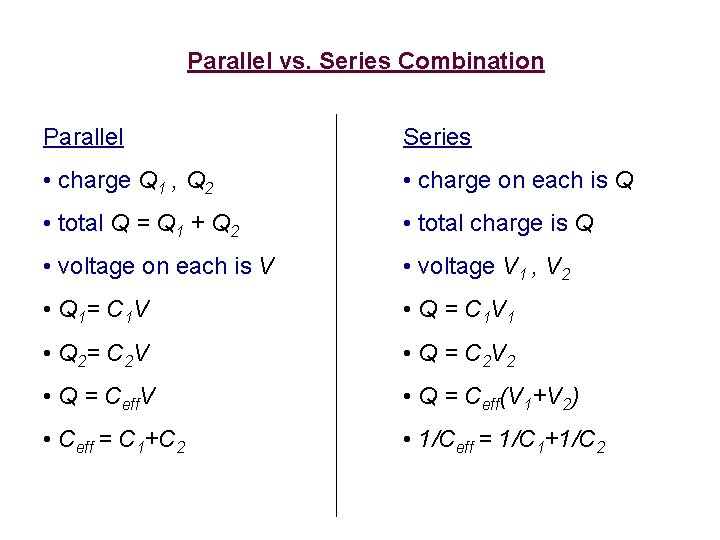Parallel vs. Series Combination Parallel Series • charge Q 1 , Q 2 • charge on each is Q • total Q = Q 1 + Q 2 • total charge is Q • voltage on each is V • voltage V 1 , V 2 • Q 1= C 1 V • Q = C 1 V 1 • Q 2= C 2 V • Q = C 2 V 2 • Q = Ceff. V • Q = Ceff(V 1+V 2) • Ceff = C 1+C 2 • 1/Ceff = 1/C 1+1/C 2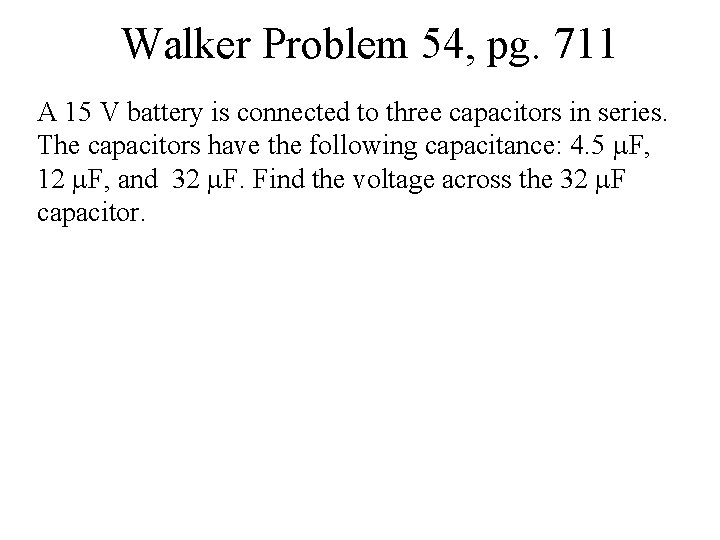Walker Problem 54, pg. 711 A 15 V battery is connected to three capacitors in series. The capacitors have the following capacitance: 4. 5 F, 12 F, and 32 F. Find the voltage across the 32 F capacitor.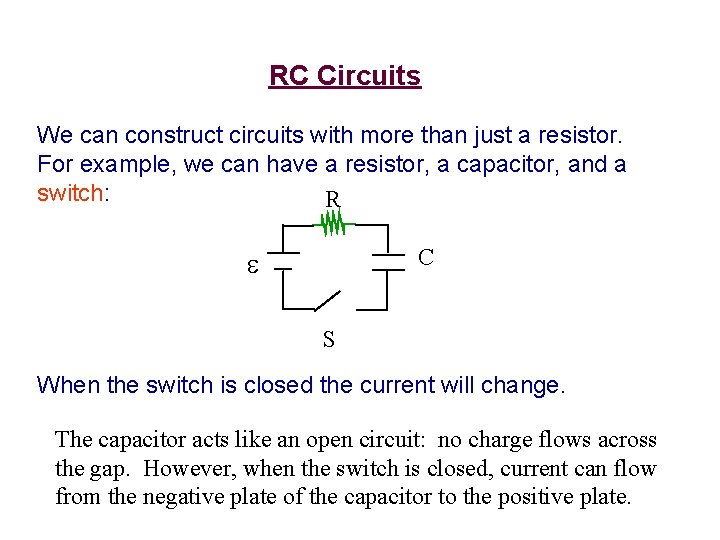RC Circuits We can construct circuits with more than just a resistor. For example, we can have a resistor, a capacitor, and a switch: R C S When the switch is closed the current will change. The capacitor acts like an open circuit: no charge flows across the gap. However, when the switch is closed, current can flow from the negative plate of the capacitor to the positive plate.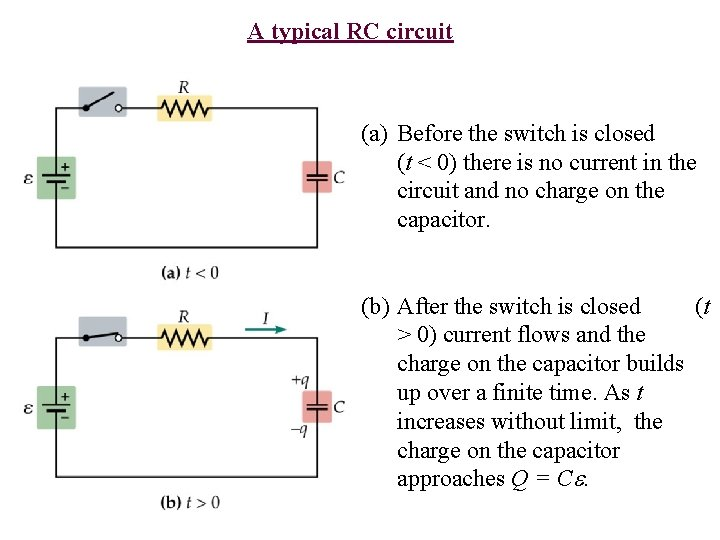A typical RC circuit (a) Before the switch is closed (t < 0) there is no current in the circuit and no charge on the capacitor. (b) After the switch is closed (t > 0) current flows and the charge on the capacitor builds up over a finite time. As t increases without limit, the charge on the capacitor approaches Q = C.Capacitor Charging Assume that at time t = 0, the capacitor is uncharged, and we close the switch. It can be shown that the charge on the capacitor at some later time t is: Charge versus time for an RC circuit q = qmax(1 – e-t/ ) The time constant = RC, and qmax is the maximum amount of charge that the capacitor will acquire: qmax=Ce The current is given by I = ( /R)e-t/ Current versus time for an RC circuitWhat happens after the switch is closed? The capacitor is initially uncharged.Walker Problem 62, pg. 712 The capacitor in an RC circuit (R = 120 , C = 45 F) is initially uncharged. Find (a) the charge on the capacitor and (b) the current in the circuit one time constant (t = RC) after the circuit is connected to a 9. 0 V battery.Walker Problem 78, pg. 713 Consider the circuit shown below. (a) Is the current flowing through the battery immediately after the switch is closed greater than, less than, or the same as the current flowing through the battery long after the switch is closed? (b) Find the current flowing through the battery immediately after the switch is closed. (c) Find the current in the battery long after the switch is closed.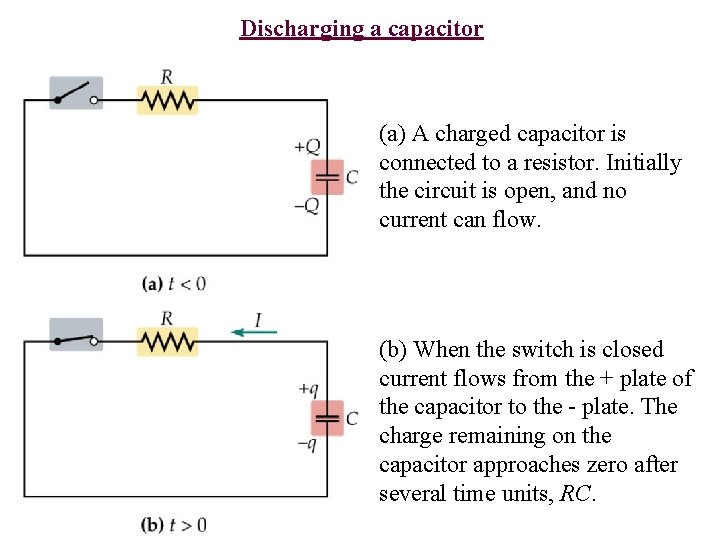Discharging a capacitor (a) A charged capacitor is connected to a resistor. Initially the circuit is open, and no current can flow. (b) When the switch is closed current flows from the + plate of the capacitor to the - plate. The charge remaining on the capacitor approaches zero after several time units, RC.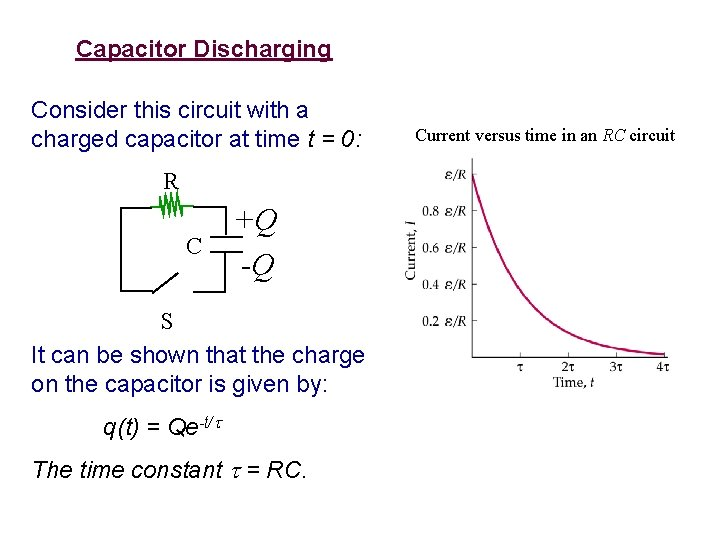Capacitor Discharging Consider this circuit with a charged capacitor at time t = 0: R C +Q -Q S It can be shown that the charge on the capacitor is given by: q(t) = Qe-t/ The time constant = RC. Current versus time in an RC circuit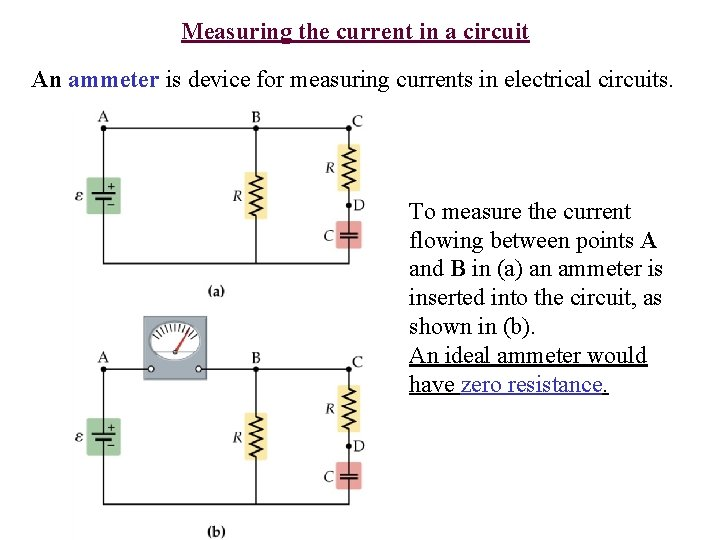Measuring the current in a circuit An ammeter is device for measuring currents in electrical circuits. To measure the current flowing between points A and B in (a) an ammeter is inserted into the circuit, as shown in (b). An ideal ammeter would have zero resistance.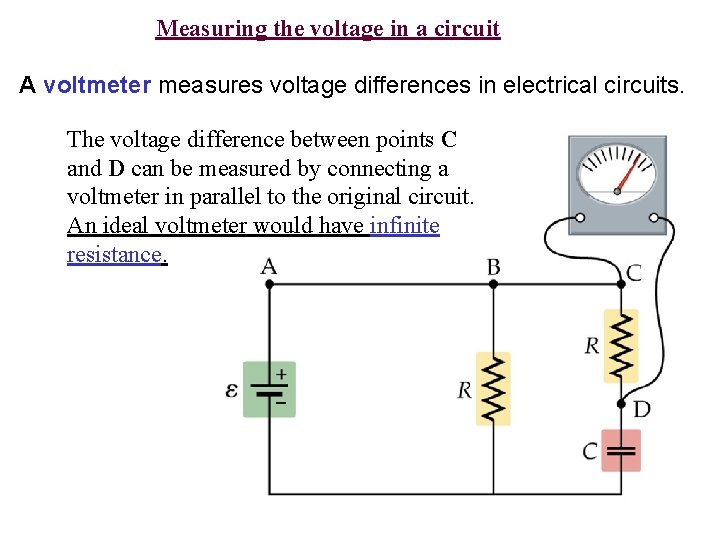Measuring the voltage in a circuit A voltmeter measures voltage differences in electrical circuits. The voltage difference between points C and D can be measured by connecting a voltmeter in parallel to the original circuit. An ideal voltmeter would have infinite resistance.